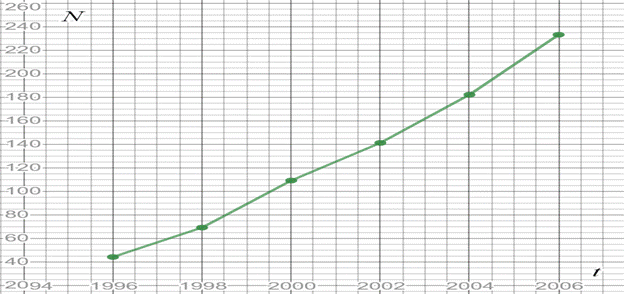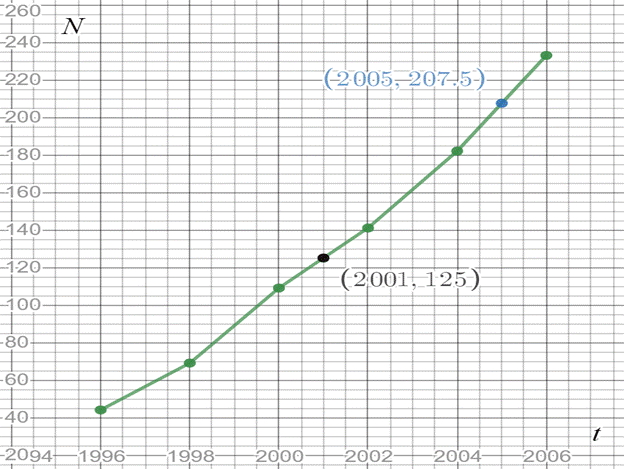# To graph : The number N ( in millions ) of US cellular phone subscribers as a function of t ( years ) with the given data is as below, t 1996 1998 2000 2002 2004 2006 N 44 69 109 141 182 233### Single Variable Calculus: Concepts...

4th Edition
James Stewart
Publisher: Cengage Learning
ISBN: 9781337687805### Single Variable Calculus: Concepts...

4th Edition
James Stewart
Publisher: Cengage Learning
ISBN: 9781337687805

#### Solutions

Chapter 1.1, Problem 21E

a.

To determine

## To graph: The number N (in millions) of US cellular phone subscribers as a function of t (years) with the given data is as below, t 199619982000200220042006 N 4469109141182233

Expert Solution

### Explanation of Solution

Given information:

The number N (in millions) of US cellular phone subscribers is shown in the table.

 t 1996 1998 2000 2002 2004 2006 N 44 69 109 141 182 233

Graph:

The graph of N vs t can be drawn by using t as x-axis and N as y-axis. The obtained graphs is as like this,Interpretation:

The obtained graph shows that the number of US cellular phone subscribers are continuously increasing from 1996 to 2006.

b.

To determine

### To calculate: The estimation of the number of cell-phone subscribers at midyear in 2001 and 2005, Using the given graph.Expert Solution

The estimated numbers of cell-phone subscribers at the midyear 2001 are 125 millions and at the midyear 2005 are 207.5 millions.

### Explanation of Solution

Given information:

The number N (in millions) of US cellular phone subscribers is shown in the table.

 t 1996 1998 2000 2002 2004 2006 N 44 69 109 141 182 233

Calculation:

Consider the given graph, the year 2001 lies in between 2000 and 2002 on the x-axis. Now, take the corresponding value of y with respect to x as 2001, it is find out to be 125 on this graph.

Similarly, the year 2005 lies in between 2004 and 2006 on the x-axis. Now, take the corresponding value of y with respect to x as 2005, it is find out to be 207.5 on this graph.Therefore, the estimated numbers of cell-phone subscribers at the midyear 2001 are 125 millions and at the midyear 2005 are 207.5 millions.

### Have a homework question?

Subscribe to bartleby learn! Ask subject matter experts 30 homework questions each month. Plus, you’ll have access to millions of step-by-step textbook answers!